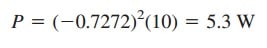# Ideal Transformers Circuit AnalysisAn ideal transformers is one with perfect coupling (k = 1). It consists of two (or more) coils with a large number of turns wound on a common core of high permeability.

Make sure to read what is linear transformer first to get the idea about how to analyze a transformer.

Contents

Because of this high permeability of the core, the flux links all the turns of both coils, thereby resulting in a perfect coupling.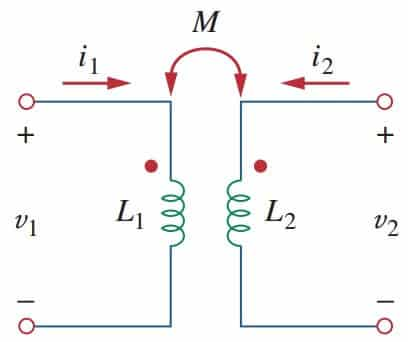## Ideal Transformers

To see how an ideal transformer is the limiting case of two coupled inductors where the inductances approach infinity and the coupling is perfect, let us reexamine the circuit in Figure.(1). In the frequency domain,

From Equation.(1a), I1 = (V1 − jωMI2)/jωL1 . Substituting this in Equation.(1b) gives

But M = √L1L2 for perfect coupling (k = 1). Hence,

where n = √L1L2 and is called the turns ratio. As L1 , L2, M → ∞ such that n remains the same, the coupled coils become an ideal transformer. A transformer is said to be ideal if it has the following properties:

1. Coils have very large reactances (L1 , L2, M → ∞).
2. The coupling coefficient is equal to unity (k = 1).
3. Primary and secondary coils are lossless (R1 = 0 = R2).

An ideal transformer is a unity-coupled, lossless transformers in which the primary and secondary coils have infinite self-inductances.

Iron-core transformers are close approximations to ideal transformers. These are used in power systems and electronics.

Figure.(2a) shows a typical ideal transformers; the circuit symbol is in Figure.(2b). The vertical lines between the coils indicate an iron core as distinct from the air core used in linear transformers. The primary winding has N1 turns; the secondary winding has N2 turns.

When a sinusoidal voltage is applied to the primary winding as shown in Figure.(3), the same magnetic flux φ goes through both windings.

According to Faraday’s law, the voltage across the primary winding is

while that across the secondary winding is

Dividing Equations.(4b) by (4a), we get

where n is, again, the turns ratio or transformation ratio. We can use the phasor voltages V1 and V2 rather than the instantaneous values v1 and v2.

Thus, Equation.(5) may be written as

For the reason of power conservation, the energy supplied to the primary must equal the energy absorbed by the secondary, since there are no losses in ideal transformers. This implies that

In phasor form, Equation.(7) in conjunction with Equation.(6) becomes

showing that the primary and secondary currents are related to the turns ratio in an inverse manner as the voltages. Thus,

When n = 1, we generally call the transformers an isolation transformers.
If n > 1, we have a step-up transformer, as the voltage is increased from primary to secondary (V2> V1).

On the other hand, if n < 1, the transformer is a step-down transformer, since the voltage is decreased from primary to secondary (V2< V1).

A step-down transformer is one whose secondary voltage is less than its primary voltage.

A step-up transformer is one whose secondary voltage is greater than its primary voltage.

The ratings of transformers are usually specified as V1/V2. A transformer with rating 2400/120 V should have 2400 V on the primary and 120 in the secondary (i.e., a step-down transformer). Keep in mind that the voltage ratings are in rms.

Power companies often generate at some convenient voltage and use a step-up transformer to increase the voltage so that the power can be transmitted at very high voltage and low current over transmission lines, resulting in significant cost savings.

Near residential consumer premises, step-down transformers are used to bring the voltage down to 120 V.

It is important that we know how to get the proper polarity of the voltages and the direction of the currents for the transformer in Figure.(3). If the polarity of V1 or V2 or the direction of I1 or I2 is changed, n in Equations.(5) to (9) may need to be replaced by −n.

The two simple rules to follow are:

1. If V1 and V2 are both positive or both negative at the dotted terminals, use +n in Equation.(6). Otherwise, use −n.
2. If I1 and I2both enter into or both leave the dotted terminals, use −n in Equation.(9). Otherwise, use +n.

The rules are demonstrated with the four circuits in Figure.(4).

Using Equations.(6) and (9), we can always express V1 in terms of V2 and I1 in terms of I2, or vice versa:

The complex power in the primary winding is

showing that the complex power supplied to the primary is delivered to the secondary without loss. The transformers absorb no power. Of course, we should expect this, since the ideal transformers are lossless.

The input impedance as seen by the source in Figure.(3) is found from Equations.(10) and (11) as

It is evident from Figure.(3) that V2/I2 = ZL, so that

The input impedance is also called the reflected impedance, since it appears as if the load impedance is reflected to the primary side.

This ability of the transformers to transform a given impedance into another impedance provides us with a means of impedance matching to ensure maximum power transfer.

In analyzing a circuit containing ideal transformers, it is common practice eliminating the transformers by reflecting impedances and sources from one side of the transformers to the other.

In the circuit of Figure.(5), suppose we want to reflect the secondary side of the circuit to the primary side. We find the Thevenin equivalent of the circuit to the right of the terminals ab.

We obtain VTh as the open-circuit voltage at terminals ab, as shown in Figure.(6a).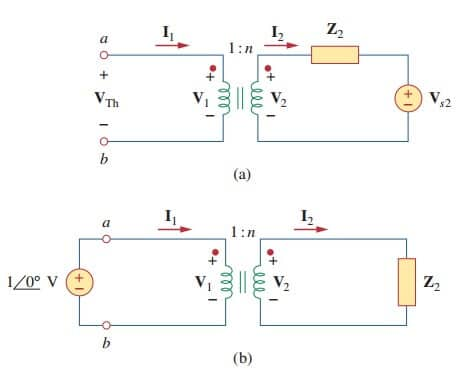Figure 6. (a) Obtaining VTh for the circuit in Figure.(5), (b) obtaining ZTh for the circuit in Figure.(5)

Since terminals ab are open, I1 = 0 = I2 so that V2 = Vs2. Hence, from Equation.(10),

To get ZTh, we remove the voltage source in the secondary winding and
insert a unit source at terminals ab, as in Figure.(6b).

From Equations.(10) and (11), I1 = nI2 and V1 = V2/n, so that

which is what we should have expected from Equation.(14). Once we have VTh and ZTh, we add the Thevenin equivalent to the part of the circuit in Figure.(5) to the left of terminals ab. Figure.(7) shows the result.Figure 7. The equivalent circuit for Figure.(5) obtained by reflecting the secondary circuit to the primary side.

The general rule for eliminating the transformer and reflecting the secondary circuit to the primary side is: divide the secondary impedance by n2, divide the secondary voltage by n, and multiply the secondary current by n.

We can also reflect the primary side of the circuit in Figure.(5) to the secondary side. Figure.(8) shows the equivalent circuit.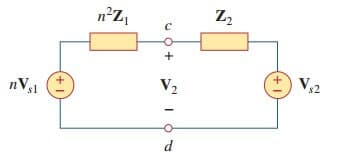Figure 8. The equivalent circuit for Figure.(5) obtained by reflecting the primary circuit to the secondary side.

The rule for eliminating the transformers and reflecting the primary circuit to the secondary side is: multiply the primary impedance by n2, multiply the primary voltage by n, and divide the primary current by n.

According to Equation.(10), the power remains the same, whether calculated on the primary or the secondary side. But realize that this reflection approach only applies if there are no external connections between the primary and secondary windings.

When we have external connections between the primary and secondary windings, we simply use regular mesh and nodal analysis.

Also, note that if the locations of the dots in Figure.(5) are changed, we might have to replace n by −n in order to obey the dot rule, illustrated in Figure.(4).

## Ideal Transformers Examples

Let’s review the ideal transformers examples below:

### Ideal Transformers Example 1

An ideal transformer is rated at 2400/120 V, 9.6 kVA, and has 50 turns on the secondary side. Calculate: (a) the turns ratio, (b) the number of turns on the primary side, and (c) the current ratings for the primary and secondary windings.

Solution:
(a) This is a step-down transformer, since V1 = 2400 V > V2 = 120 V.(b)or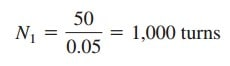(c) S = V1I1 = V2I2 = 9.6 kVA. Hence,### Ideal Transformers Example 2

For the ideal transformer circuit of Figure.(2.1), find: (a) the source current I1, (b) the output voltage Vo, and (c) the complex power supplied by the source.(a) The 20-Ω impedance can be reflected to the primary side and we get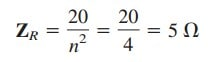Thus,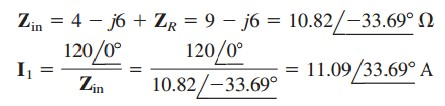(b) Since both I1 and I2 leave the dotted terminals,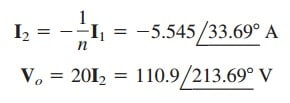(c) The complex power supplied is### Ideal Transformers Example 3

Calculate the power supplied to the 10-Ω resistor in the ideal transformer circuit of the figure below.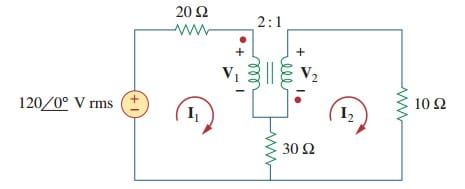Reflection to the secondary or primary side cannot be done with this circuit: there is a direct connection between the primary and secondary sides due to the 30-Ω resistor. We apply mesh analysis. For mesh 1,or

For mesh 2,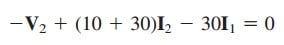or

At the transformer terminals,

(Note that n = 1/2.) We now have four equations and four unknowns, but our goal is to get I2. So we substitute for V1 and I1 in terms of V2 and I2 in Equations.(3.1) and (3.2).

Equation (3.1) becomes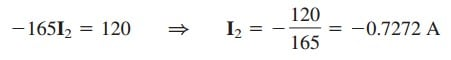The power absorbed by the 10-Ω resistor is